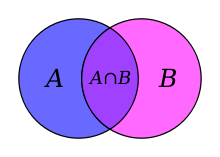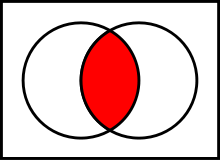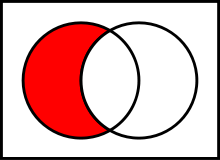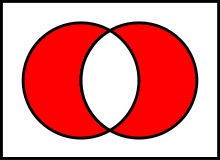# Set (mathematics)

﻿
Set (mathematics)The intersection of two sets is made up of the objects contained in both sets, shown in a Venn diagram.

A set is a collection of well defined and distinct objects, considered as an object in its own right. Sets are one of the most fundamental concepts in mathematics. Developed at the end of the 19th century, set theory is now a ubiquitous part of mathematics, and can be used as a foundation from which nearly all of mathematics can be derived. In mathematics education, elementary topics such as Venn diagrams are taught at a young age, while more advanced concepts are taught as part of a university degree.

## Definition

Georg Cantor, the founder of set theory, gave the following definition of a set at the beginning of his Beiträge zur Begründung der transfiniten Mengenlehre:

A set is a gathering together into a whole of definite, distinct objects of our perception [Anschauung] and of our thought – which are called elements of the set.

The elements or members of a set can be anything: numbers, people, letters of the alphabet, other sets, and so on. Sets are conventionally denoted with capital letters. Sets A and B are equal if and only if they have precisely the same elements.

As discussed below, the definition given above turned out to be inadequate for formal mathematics; instead, the notion of a "set" is taken as an undefined primitive in axiomatic set theory, and its properties are defined by the Zermelo–Fraenkel axioms. The most basic properties are that a set "has" elements, and that two sets are equal (one and the same) if and only if they have the same elements.

## Describing sets

There are two ways of describing, or specifying the members of, a set. One way is by intensional definition, using a rule or semantic description:

A is the set whose members are the first four positive integers.
B is the set of colors of the French flag.

The second way is by extension – that is, listing each member of the set. An extensional definition is denoted by enclosing the list of members in curly brackets:

C = {4, 2, 1, 3}
D = {blue, white, red}.

Every element of a set must be unique; no two members may be identical. (A multiset is a generalized concept of a set that that relaxes this criterion.) All set operations preserve this property. The order in which the elements of a set or multiset are listed is irrelevant (unlike for a sequence or tuple). Combining these two ideas into an example

{6, 11} = {11, 6} = {11, 11, 6, 11}

because the extensional specification means merely that each of the elements listed is a member of the set.

For sets with many elements, the enumeration of members can be abbreviated. For instance, the set of the first thousand positive integers may be specified extensionally as:

{1, 2, 3, ..., 1000},

where the ellipsis ("...") indicates that the list continues in the obvious way. Ellipses may also be used where sets have infinitely many members. Thus the set of positive even numbers can be written as {2, 4, 6, 8, ... }.

The notation with braces may also be used in an intensional specification of a set. In this usage, the braces have the meaning "the set of all ...". So, E = {playing card suits} is the set whose four members are ♠, ♦, ♥, and ♣. A more general form of this is set-builder notation, through which, for instance, the set F of the twenty smallest integers that are four less than perfect squares can be denoted:

F = {n2 − 4 : n is an integer; and 0 ≤ n ≤ 19}.

In this notation, the colon (":") means "such that", and the description can be interpreted as "F is the set of all numbers of the form n2 − 4, such that n is a whole number in the range from 0 to 19 inclusive." Sometimes the vertical bar ("|") is used instead of the colon.

One often has the choice of specifying a set intensionally or extensionally. In the examples above, for instance, A = C and B = D.

## Membership

The key relation between sets is membership – when one set is an element of another. If a is a member of B, this is denoted aB, while if c is not a member of B then cB. For example, with respect to the sets A = {1,2,3,4}, B = {blue, white, red}, and F = {n2 − 4 : n is an integer; and 0 ≤ n ≤ 19} defined above,

4 ∈ A and 285 ∈ F; but
9 ∉ F and green ∉ B.

### Subsets

If every member of set A is also a member of set B, then A is said to be a subset of B, written AB (also pronounced A is contained in B). Equivalently, we can write BA, read as B is a superset of A, B includes A, or B contains A. The relationship between sets established by ⊆ is called inclusion or containment.

If A is a subset of, but not equal to, B, then A is called a proper subset of B, written AB (A is a proper subset of B) or BA (B is a proper superset of A).

Note that the expressions AB and BA are used differently by different authors; some authors use them to mean the same as AB (respectively BA), whereas other use them to mean the same as AB (respectively BA).

Example:

• The set of all men is a proper subset of the set of all people.
• {1, 3} ⊊ {1, 2, 3, 4}.
• {1, 2, 3, 4} ⊆ {1, 2, 3, 4}.

The empty set is a subset of every set and every set is a subset of itself:

• ∅ ⊆ A.
• AA.

An obvious but useful identity, which can often be used to show that two seemingly different sets are equal:

• A = B if and only if AB and BA.

### Power sets

The power set of a set S is the set of all subsets of S. This includes the subsets formed from all the members of S and the empty set. If a finite set S has cardinality n then the power set of S has cardinality 2n. The power set of S can be written as P(S).

If S is an infinite (either countable or uncountable) set, then the power set of S is always uncountable. Moreover, if S is a set, then there is never a bijection from S onto P(S). In other words, the power set of S is always strictly "bigger" than S.

As an example, the power set of {1, 2, 3} is {{1, 2, 3}, {1, 2}, {1, 3}, {2, 3}, {1}, {2}, {3}, ∅}. The cardinality of the original set is 3, and the cardinality of the power set is 23 = 8. This relationship is one of the reasons for the terminology power set.

## Cardinality

The cardinality | S | of a set S is "the number of members of S." For example, if B = {blue, white, red}, | B | = 3.

There is a unique set with no members and zero cardinality, which is called the empty set (or the null set) and is denoted by the symbol ∅ (other notations are used; see empty set). For example, the set of all three-sided squares has zero members and thus is the empty set. Though it may seem trivial, the empty set, like the number zero, is important in mathematics; indeed, the existence of this set is one of the fundamental concepts of axiomatic set theory.

Some sets have infinite cardinality. The set N of natural numbers, for instance, is infinite. Some infinite cardinalities are greater than others. For instance, the set of real numbers has greater cardinality than the set of natural numbers. However, it can be shown that the cardinality of (which is to say, the number of points on) a straight line is the same as the cardinality of any segment of that line, of the entire plane, and indeed of any finite-dimensional Euclidean space.

## Special sets

There are some sets which hold great mathematical importance and are referred to with such regularity that they have acquired special names and notational conventions to identify them. One of these is the empty set, denoted {} or ∅. Another is the unit set {x} which contains exactly one element, namely x. Many of these sets are represented using blackboard bold or bold typeface. Special sets of numbers include:

• P or ℙ, denoting the set of all primes: P = {2, 3, 5, 7, 11, 13, 17, ...}.
• N or ℕ, denoting the set of all natural numbers: N = {1, 2, 3, . . .}.
• Z or ℤ, denoting the set of all integers (whether positive, negative or zero): Z = {... , −2, −1, 0, 1, 2, ...}.
• Q or ℚ, denoting the set of all rational numbers (that is, the set of all proper and improper fractions): Q = {a/b : a, bZ, b ≠ 0}. For example, 1/4 ∈ Q and 11/6 ∈ Q. All integers are in this set since every integer a can be expressed as the fraction a/1 (ZQ).
• R or ℝ, denoting the set of all real numbers. This set includes all rational numbers, together with all irrational numbers (that is, numbers which cannot be rewritten as fractions, such as π, e, and √2, as well as numbers that cannot be defined).
• C or ℂ, denoting the set of all complex numbers: C = {a + bi : a, bR}. For example, 1 + 2iC.
• H or ℍ, denoting the set of all quaternions: H = {a + bi + cj + dk : a, b, c, dR}. For example, 1 + i + 2jkH.

Each of the above sets of numbers has an infinite number of elements, and each can be considered to be a proper subset of the sets listed below it. The primes are used less frequently than the others outside of number theory and related fields.

## Basic operations

There are several fundamental operations for constructing new sets from given sets.

### UnionsThe union of A and B, denoted AB

Two sets can be "added" together. The union of A and B, denoted by A ∪ B, is the set of all things which are members of either A or B.

Examples:

• {1, 2} ∪ {red, white} ={1, 2, red, white}.
• {1, 2, green} ∪ {red, white, green} ={1, 2, red, white, green}.
• {1, 2} ∪ {1, 2} = {1, 2}.

Some basic properties of unions:

• AB = BA.
• A ∪ (BC) = (AB) ∪ C.
• A ⊆ (AB).
• AB if and only if AB = B.
• AA = A.
• A ∪ ∅ = A.

### Intersections

A new set can also be constructed by determining which members two sets have "in common". The intersection of A and B, denoted by AB, is the set of all things which are members of both A and B. If AB = ∅, then A and B are said to be disjoint.The intersection of A and B, denoted AB.

Examples:

• {1, 2} ∩ {red, white} = ∅.
• {1, 2, green} ∩ {red, white, green} = {green}.
• {1, 2} ∩ {1, 2} = {1, 2}.

Some basic properties of intersections:

• AB = BA.
• A ∩ (BC) = (AB) ∩ C.
• ABA.
• AA = A.
• A ∩ ∅ = ∅.
• AB if and only if AB = A.

### ComplementsThe relative complement
of B in AThe complement of A in UThe symmetric difference of A and B

Two sets can also be "subtracted". The relative complement of B in A (also called the set-theoretic difference of A and B), denoted by A \ B (or AB), is the set of all elements which are members of A but not members of B. Note that it is valid to "subtract" members of a set that are not in the set, such as removing the element green from the set {1, 2, 3}; doing so has no effect.

In certain settings all sets under discussion are considered to be subsets of a given universal set U. In such cases, U \ A is called the absolute complement or simply complement of A, and is denoted by A′.

Examples:

• {1, 2} \ {red, white} = {1, 2}.
• {1, 2, green} \ {red, white, green} = {1, 2}.
• {1, 2} \ {1, 2} = ∅.
• {1, 2, 3, 4} \ {1, 3} = {2, 4}.
• If U is the set of integers, E is the set of even integers, and O is the set of odd integers, then E′ = O.

Some basic properties of complements:

• A \ BB \ A.
• AA′ = U.
• AA′ = ∅.
• (A′)′ = A.
• A \ A = ∅.
• U′ = ∅ and ∅′ = U.
• A \ B = AB.

An extension of the complement is the symmetric difference, defined for sets A, B as$A\,\Delta\,B = (A \setminus B) \cup (B \setminus A).$

For example, the symmetric difference of {7,8,9,10} and {9,10,11,12} is the set {7,8,11,12}.

### Cartesian product

A new set can be constructed by associating every element of one set with every element of another set. The Cartesian product of two sets A and B, denoted by A × B is the set of all ordered pairs (a, b) such that a is a member of A and b is a member of B.

Examples:

• {1, 2} × {red, white} = {(1, red), (1, white), (2, red), (2, white)}.
• {1, 2, green} × {red, white, green} = {(1, red), (1, white), (1, green), (2, red), (2, white), (2, green), (green, red), (green, white), (green, green)}.
• {1, 2} × {1, 2} = {(1, 1), (1, 2), (2, 1), (2, 2)}.

Some basic properties of cartesian products:

• A × ∅ = ∅.
• A × (BC) = (A × B) ∪ (A × C).
• (AB) × C = (A × C) ∪ (B × C).

Let A and B be finite sets. Then

• | A × B | = | B × A | = | A | × | B |.

## Applications

Set theory is seen as the foundation from which virtually all of mathematics can be derived. For example, structures in abstract algebra, such as groups, fields and rings, are sets closed under one or more operations.

One of the main applications of naive set theory is constructing relations. A relation from a domain A to a codomain B is a subset of the Cartesian product A × B. Given this concept, we are quick to see that the set F of all ordered pairs (x, x2), where x is real, is quite familiar. It has a domain set R and a codomain set that is also R, because the set of all squares is subset of the set of all reals. If placed in functional notation, this relation becomes f(x) = x2. The reason these two are equivalent is for any given value, y that the function is defined for, its corresponding ordered pair, (y, y2) is a member of the set F.

## Axiomatic set theory

Although initially naive set theory, which defines a set merely as any well-defined collection, was well accepted, it soon ran into several obstacles. It was found that this definition spawned several paradoxes, most notably:

• Russell's paradox—It shows that the "set of all sets which do not contain themselves," i.e. the "set" { x : x is a set and xx } does not exist.
• Cantor's paradox—It shows that "the set of all sets" cannot exist.

The reason is that the phrase well-defined is not very well defined. It was important to free set theory of these paradoxes because nearly all of mathematics was being redefined in terms of set theory. In an attempt to avoid these paradoxes, set theory was axiomatized based on first-order logic, and thus axiomatic set theory was born.

For most purposes however, naive set theory is still useful.

## Principle of inclusion and exclusion

This principle gives us the cardinality of intersection of sets.

|A1 ∩ A2 ∩ A3 ∩ A4 ∩ ....... ....∩ An|=(|A1| + |A2| + |A3| +...+ |An|)-(|A1 ∩ A2| +|A2 ∩ A3| + ....+|An-1 ∩ An|) + .........+(−1)n-1(|A1 ∩ A2 ∩ A3 ∩.....∩ An|)

Wikimedia Foundation. 2010.

### Look at other dictionaries:

• Derived set (mathematics) — In mathematics, more specifically in point set topology, the derived set of a subset S of a topological space is the set of all limit points of S. It is usually denoted by S′. The concept was first introduced by Georg Cantor in 1872 and he… …   Wikipedia

• Set — has 464 separate definitions in the Oxford English Dictionary, the most of any English word; its full definition comprises 10,000 words making it the longest definition in the OED. Set may refer to:In mathematics and science:*Set (mathematics), a …   Wikipedia

• Set theory — This article is about the branch of mathematics. For musical set theory, see Set theory (music). A Venn diagram illustrating the intersection of two sets. Set theory is the branch of mathematics that studies sets, which are collections of objects …   Wikipedia

• Mathematics — Maths and Math redirect here. For other uses see Mathematics (disambiguation) and Math (disambiguation). Euclid, Greek mathematician, 3r …   Wikipedia

• Mathematics and art — have a long historical relationship. The ancient Egyptians and ancient Greeks knew about the golden ratio, regarded as an aesthetically pleasing ratio, and incorporated it into the design of monuments including the Great Pyramid, the Parthenon …   Wikipedia

• Mathematics, Form and Function — is a survey of the whole of mathematics, including its origins and deep structure, by the American mathematician Saunders Mac Lane. Contents 1 Mac Lane s relevance to the philosophy of mathematics 2 Mathematics and human activities …   Wikipedia

• Set (game) — Set! redirects here. Set! is also a special form in the Scheme programming language. Set is a real time card game designed by Marsha Falco and published by Set Enterprises in 1991. The deck consists of 81 cards varying in four features: number… …   Wikipedia

• Mathematics (producer) — Mathematics At the Eurockéennes of 2007. Background information Also known as Allah Mathematics Origin …   Wikipedia

• set — /set/, v., set, setting, n., adj., interj. v.t. 1. to put (something or someone) in a particular place: to set a vase on a table. 2. to place in a particular position or posture: Set the baby on his feet. 3. to place in some relation to something …   Universalium

• set — Ⅰ. set  ► VERB (setting; past and past part. set) 1) put, lay, or stand in a specified place or position. 2) put, bring, or place into a specified state. 3) cause or instruct (someone) to do something. 4) give someone (a task) …   English terms dictionary